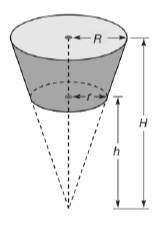Chapter 9.3, Problem 44EElementary Geometry For College St...

7th Edition
Alexander + 2 others
ISBN: 9781337614085

Solutions

Chapter
SectionElementary Geometry For College St...

7th Edition
Alexander + 2 others
ISBN: 9781337614085
Textbook Problem

In Exercises 44 and 45, use the formula from Exercise 43. Similar triangles were used to find h and H.A margarine tub has the shape of a frustum of a cone. With the lower base having diameter length 11 cm and the upper base having diameter length 14 cm, the volume of such a container 6 2 3 cm tall can be determine by using H = 32 2 3 cm, h = 26 cm. Find its volume.A frustum of a cone is the portion of the cone bounded between the circular base and a plane parallel to the base. With dimensions are indicated, show that the volume of the frustum of the cone isV = 1 3 π R 2 H − 1 3 π r h 2

To determine

To find:

The volume of the margarine tub.

Explanation

Given:

A margarine tub has the shape of the frustrum of a cone.With the lower base having diameter 11 cm and the upper base having diameter 14 cm, the volume of such a container 623 cm tall can be determined by using H=3223 cm, h=26 cm.

Formula used:

The volume of the frustum of the cone is V=13πR2H13πr2h.

Calculation:

We have r=5.5 cm, h=26 cm and R=7 cm, H=32.67 cm

Still sussing out bartleby?

Check out a sample textbook solution.

See a sample solution

The Solution to Your Study Problems

Bartleby provides explanations to thousands of textbook problems written by our experts, many with advanced degrees!

Get Started